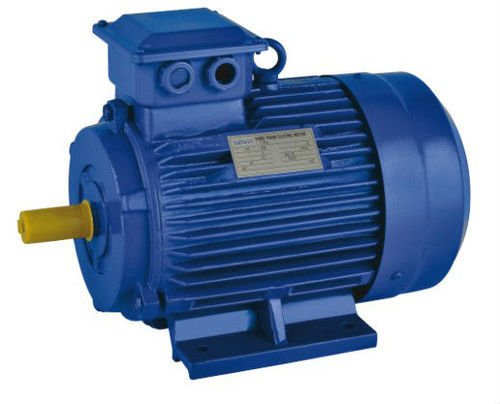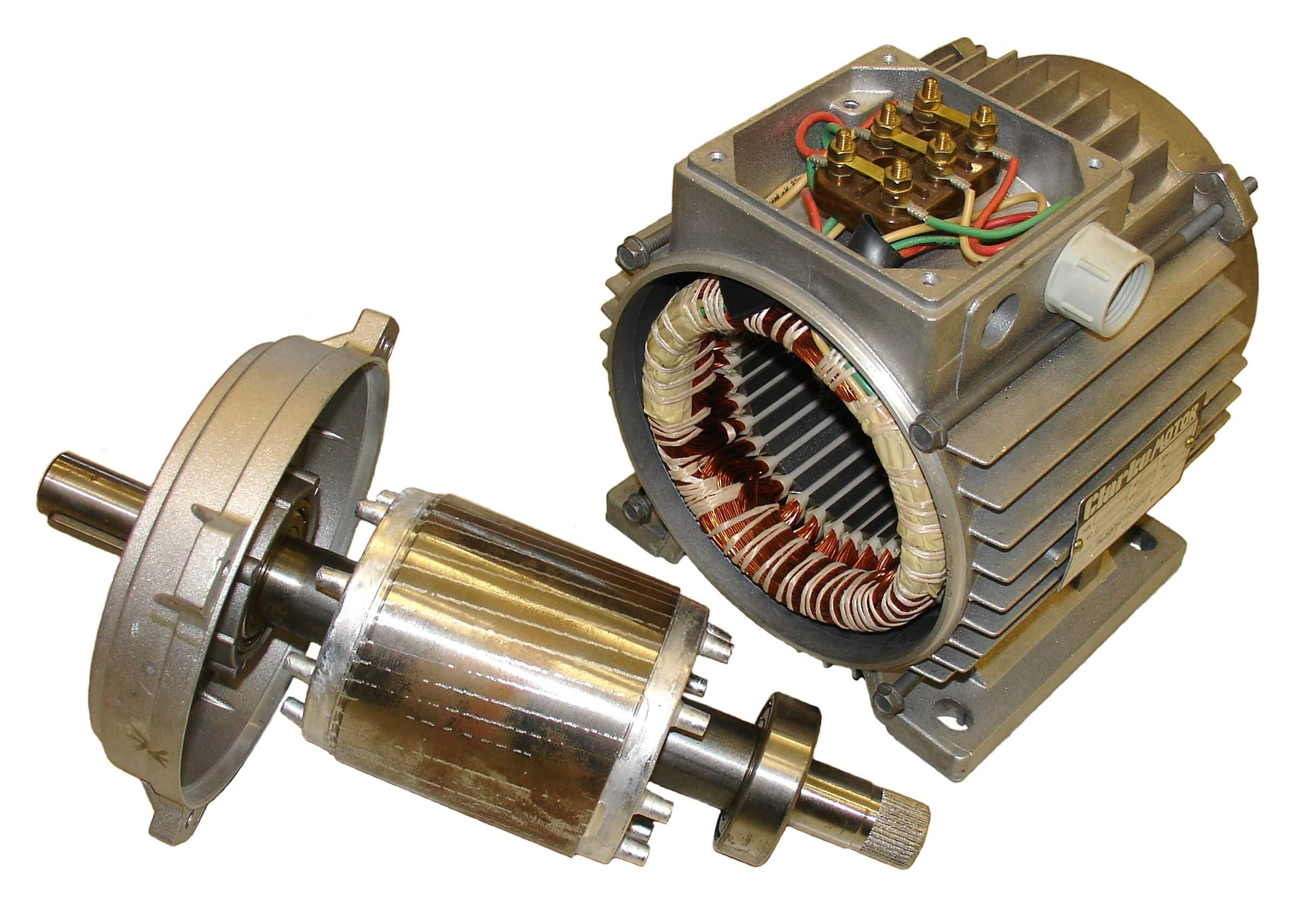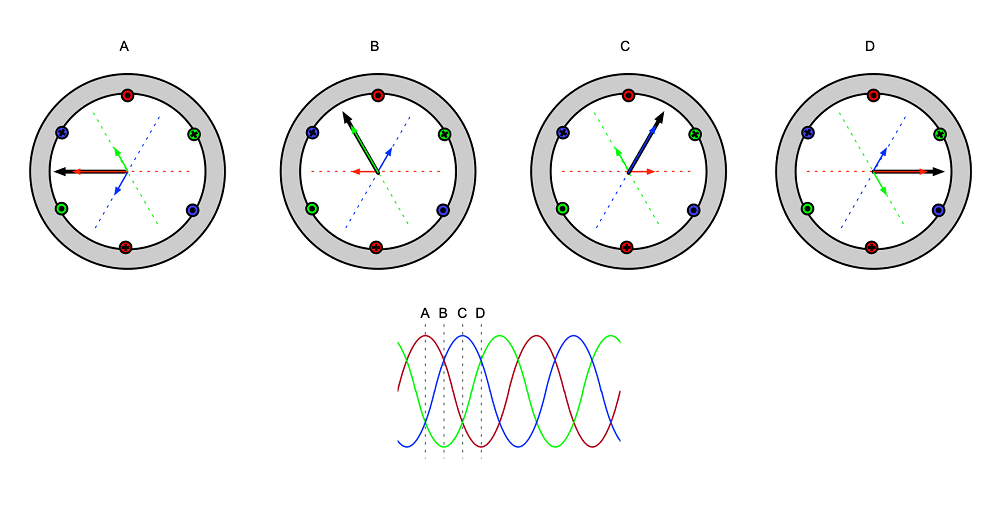# Induction MotorsWritten by

Induction motors are by far the most common type of electric motor in use today. This is because they are simple in construction, relatively cheap to produce and extremely reliable, given they effectively have just one moving part. Unlike DC motors, or universal motors, no brushes or sliding contacts are required to transfer energy to the rotor—this happened by induction as we will see. Unlike permanent magnet motors or brushless DC (BLDC) motors, no exotic magnets or Hall-effect rotor position sensors are required.

Let’s start by looking at the typical three-phase induction motor commonly used in industrial settings. This illustrates the principles of operation that apply to the other types. The stationary part of the motor, the stator, carries three windings, each connected to a different phase of the incoming supply. These are arranged in an interleaved manner, so they create a rotating magnetic field. Figure 1 shows a disassembled motor. You can just see the stator windings on the right-hand side, running parallel to the axis of the motor in the stator slots under strips of insulating material.FIGURE 1. This photo shows how a typical small three-phase induction motor is built. The stator windings run in slots in the stator parallel to the axis of the motor. The rotor is made from steel laminations with rotor “windings” in the form of aluminum bars embedded in them. You can just see the top of the bars running at a slight angle to the axis of the stator. The bars are shorted by aluminum rings visible at the ends of the rotor stack.

Figure 2 shows a simplified cross-section of the stator, which is made of many thin laminations of steel, just like a mains transformer. The windings are represented in the diagram by the red, green, and blue circles. A dot in the circle denotes positive current flowing away from us, and a cross denotes it flowing towards us. A current flowing in a winding will create a magnetic field at a right angle to the winding along the relevant axis shown dotted. The size and direction of this field will depend on the polarity and magnitude of the current at any point in time.FIGURE 2. This simplified diagram shows how the three-phase current produces a rotating flux vector. Each “snapshot” is taken at a point one sixth of a cycle later than the previous one. The individual windings produce a magnetic field with an angle and magnitude dependent on the polarity and magnitude of the supply current. These add to give a net flux vector shown by the heavy black arrow. With this arrangement of windings, the flux vector rotates smoothly around the stator once for every mains (power grid) cycle.

At point A in the mains cycle, for example, the red phase current will be at its maximum positive value and the green and blue currents will be negative and a little smaller in magnitude. The fields they produce are shown as vectors (arrows) of the appropriate color. The net magnetic field will therefore point to the left as shown by the heavy black vector.  At point B in the waveform (one sixth of a cycle or 60° later) the green phase current will be at its maximum negative excursion and the red and blue phase currents will be positive but with slightly smaller magnitude. This results in a net flux vector as shown at B.

It works similarly for point C and so on. The three-phase winding produces a magnetic field that rotates smoothly around the stator once for each cycle of the mains. This corresponds to a rotational speed of 50 revolutions per second, or 3000 rpm for 50Hz mains and 3600 rpm for 60Hz mains. This arrangement is known as a 2-pole configuration. since there are 2 poles (north & south) of each phase winding.

If we interleave another set windings as shown in Figure 3 the same analysis shows the net flux vector now takes two mains (power grid) cycles to rotate around the stator. Our flux vector now rotates at 1500 rpm or 1800 rpm depending on the mains frequency. This is known as a 4-pole configuration. small induction motors such as this are commonly available in 2, 4, 6 or (rarely) 8-pole configurations. The speed of the rotating flux vector is known as the synchronous speed (ns) of the induction motor. The shaft does not rotate at this speed as we shall see.FIGURE 3. Interleaving a second set of windings as shown here produces a flux vector which rotates at half the speed of that in Figure 2. This configuration is known as a four-pole motor. The field rotates at a synchronous speed of 1500 rpm for 50 Hz mains and 1800 rpm for 60 Hz.

Now for the awesome piece. If we insert a rotor which also has a set of windings on it, we will get a current flowing in these windings by transformer action. You can see from Figure 1 that the rotor is also made of laminated steel and the “windings” are usually aluminum bars cast into slots and short-circuited by an aluminum ring at each end. If you can visualize the rotor “windings” without the laminated steel core you will understand why this type of motor is often referred to as a squirrel cage induction motor. Figure 4 shows this in simplified form.FIGURE 4. This diagram shows the same winding configuration as Figure 2, but with a rotor in place. The rotating magnetic field induces current in the stator by transformer action. This current produces its own magnetic field which interacts with the stator field to produce a torque which accelerates the rotor. As the rotor increases in speed, the relative rotational speed of the stator flux vector seen by the rotor, and therefore the torque, reduces. The stator settles into a stable no-load speed when this torque is balanced by the friction and windage of the rotor.

When the rotor is stationary, the currents induced in the rotor bars can be very large, since they are effectively shorted turns. The rotor current will be at the supply frequency at this point. The rotor currents produce a magnetic field that interacts with the stator field and so a torque is created and the stator to begin to rotate.

As the rotor increases in speed, the frequency and amplitude of the current induced in the stator bars reduces. This is because the speed of the rotating flux seen by the rotor relative to the stator is reduces as the rotor accelerates toward the synchronous speed.

If the rotor was able to reach the synchronous speed, there would be no induced rotor current and no torque, since the rotor would see the stator flux vector as stationary, and we know induction can only occur with a changing magnetic field.

The induction motor at no load, therefore, settles into a speed just a little lower than the synchronous speed, where the diminishing torque produced by the rotor-stator field interaction is balanced by the torque required to overcome friction and windage (the drag from the air in the rotor). The difference between the synchronous speed and the rotor speed is known as the slip which is measured in percent:

Typical no-load slip for an induction motor is just a few percent, so the no-load speed of a typical 4-pole motor at 50 Hz might be around 1,450 rpm. As the motor is loaded, the slip increases, and the motor slows down slightly. As this happens the current induced in the rotor increases in both magnitude and frequency providing more reaction against the rotating field and more torque. The relationship between torque and speed for a typical induction motor is shown in Figure 5.FIGURE 5. This graph shows the torque-speed characteristic of an induction motor in red. The torque is zero at the synchronous speed ns. The motor operates at a no-load point A, where the rotor speed is just below the synchronous speed. As the motor is loaded, the slip increases, and the rotor speed reduces slightly. Steady state operation is achieved at the intersection of the motor and load torque-speed curves as shown at points B and C. Pont D is the “pull-out torque” where the motor cannot provide sufficient torque to drive the load.

The red line represents the motor torque-speed characteristic. You can see that at the synchronous speed ns the torque is zero. The operating range of the motor is the almost vertical region on the right of the curve. The no-load operating point is labelled point A – here the slip is small, and the rotational speed is close to the synchronous speed.

Loke all motors, the operating point will be the intersection of the motor’s torque-speed characteristic and that of the load. Two such load characteristics are shown on the graph, a constant torque such as a conveyor load in green and a quadratic load such as a fan in blue. The operating points of these loads are labelled B and C respectively. The pull-out torque (point D) is the torque at which the motor can no longer drive the load and will stall

At full load the slip for a typical motor will be in the range 5 to 10% so the speed regulation over the load range will be similar. Induction therefore motors do not have perfect speed regulation like a synchronous motor, but more than make up for this deficiency in terms of simplicity, cost and reliability.

There is a lot more to explore when it comes to induction motors—for example we have not touched on single-phase induction motors nor how we can control and regulate the speed of these motors. I might cover these topics in future articles.

Reference:
“Induction Motor.” In Wikipedia, October 6, 2021. https://en.wikipedia.org/w/index.php?title=Induction_motor&oldid=1048471037

 Keep up-to-date with our FREE Weekly Newsletter! Don't miss out on upcoming issues of Circuit Cellar. Subscribe to Circuit Cellar Magazine Note: We’ve made the May 2020 issue of Circuit Cellar available as a free sample issue. In it, you’ll find a rich variety of the kinds of articles and information that exemplify a typical issue of the current magazine. Would you like to write for Circuit Cellar? We are always accepting articles/posts from the technical community. Get in touch with us and let's discuss your ideas.